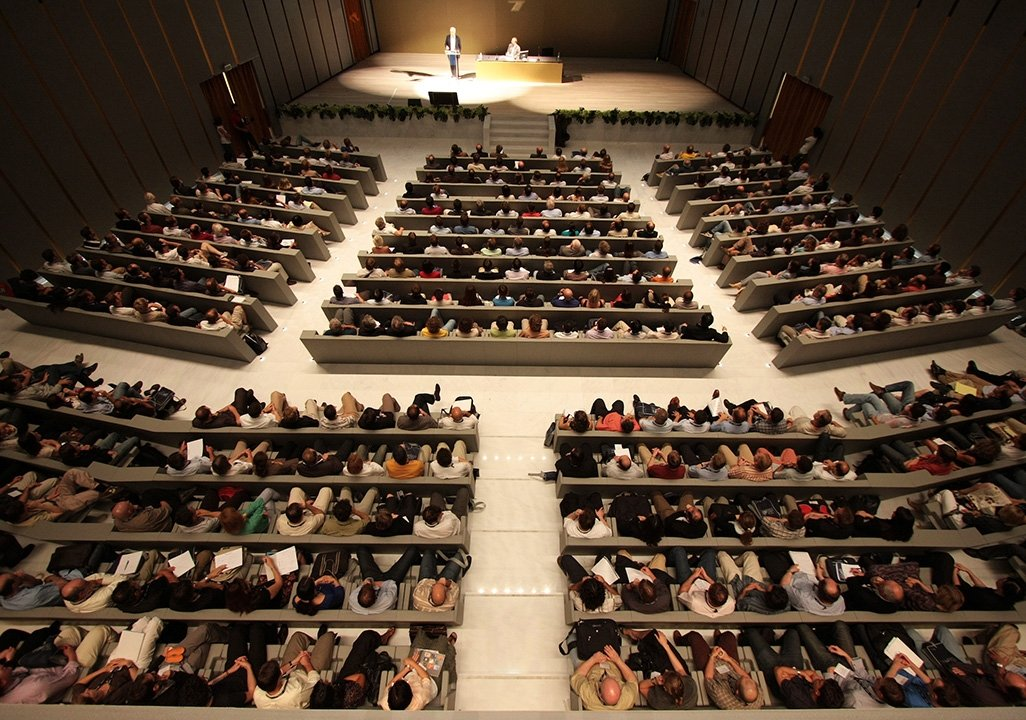## Dario Trevisan: Gaussian Processes as Approximations of Random Neural Networks

Seminars - Analysis and Applied Mathematics Seminar
Speakers
DARIO TREVISAN, Universita' di Pisa
12:30 - 14:51
Room 3-E4-SR03Abstract:

Deep neural networks are commonly initialized with random parameters from Gaussian distributions. This talk analyzes the relationship between the output distribution of such a randomly initialized neural network and an equivalent Gaussian process distribution. Explicit inequalities are derived bounding the quadratic Wasserstein distance between the network outputs and a Gaussian distribution as a function of the network architecture. As the hidden layer sizes increase, the bounds quantify how the network output distribution converges to a Gaussian in the so-called "wide limit". Furthermore, the bounds can be extended to characterize the Gaussian approximation of the exact Bayesian posterior distribution over the weights. The results provide a quantitative mathematical understanding of when and why random neural networks exhibit Gaussian-like behavior, with implications for modeling and analysis in machine learning applications. Joint work with A. Basteri (arXiv:2203.07379).